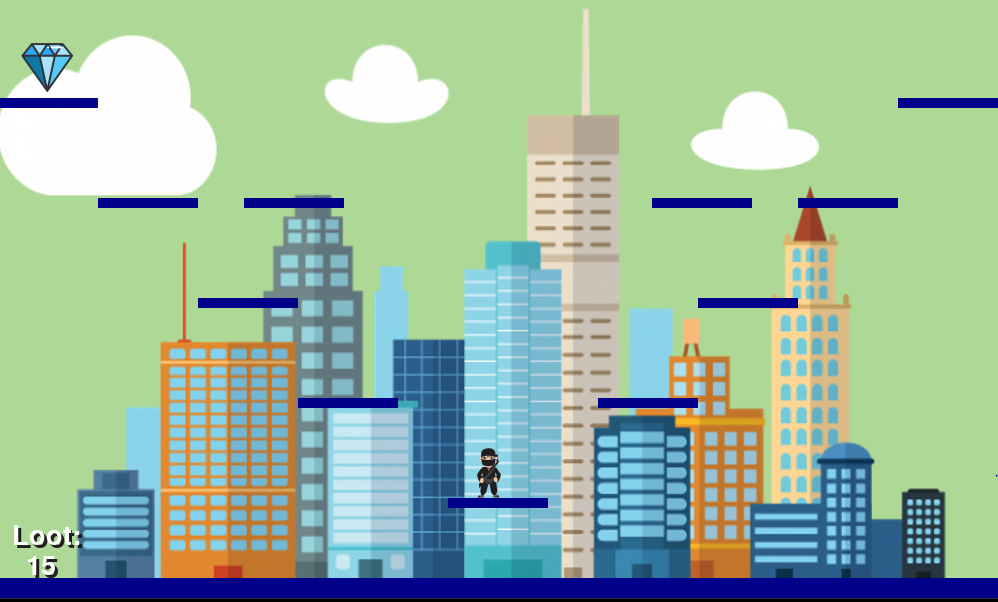# Welcome to my ninja jumper game in python!

I am Lluis. In this webpage you will find my codes using Python.

This game consists of moving the ninja to get the gems and the maximum score by jumping over differents platforms usings the keyboard arrows

This is my python code:

``````
# NINJA JUMPER
import time
import pygame
import random

WIDTH = 1000
HEIGHT = 600
blue = 150
blueforward = True
groundcolour = 0, 0, 139
floor = Rect((0, 580), (1000, 20))
# Ninja
ninja = Actor("jumper-1", (500, 250))
ninja_x_velocity = 0
ninja_y_velocity = 0
gravity = 1
jumping = False
jumped = False
allowx = True
timer = []
# platforms
platform1 = Rect((450, 500), (100, 10))
platform2 = Rect((300, 400), (100, 10))
platform3 = Rect((600, 400), (100, 10))
platform4 = Rect((200, 300), (100, 10))
platform5 = Rect((700, 300), (100, 10))
platform6 = Rect((100, 200), (100, 10))

plat61_x = 200
plat62_x = 700
platform61 = Rect((plat61_x, 200), (100, 10))
platform62 = Rect((plat62_x, 200), (100, 10))
platform7 = Rect((800, 200), (100, 10))  # ..moving out right(
platform8 = Rect((0, 100), (100, 10))  # top far left
platform9 = Rect((900, 100), (100, 10))  # top far rigth
platforms = [
floor,
platform1,
platform2,
platform3,
platform4,
platform5,
platform6,
platform7,
platform8,
platform9,
platform61,
platform62,
]  # list to platforms
plat61left = True
plat62left = False
# Gems
diamond_x = [950, 50, 850, 150, 750, 250, 650, 350, 500]
diamond_y = [70, 70, 170, 170, 270, 270, 370, 370, 470]
d_xy = random.randint(0, 8)
gem = Actor("diamond_s", (diamond_x[d_xy], diamond_y[d_xy]))
points = 0
# music
pygame.mixer.pre_init(22050, -16, 2, 1024)
pygame.init()
pygame.mixer.quit()
pygame.mixer.init(22050, -16, 2, 1024)
sounds.ninja_music.play(-1)

def draw():
global platform61, platform62
screen.fill((173, 216, blue))
screen.blit("skyline_large", (0, 0))
platform61 = Rect((plat61_x, 200), (100, 10))
platform62 = Rect((plat62_x, 200), (100, 10))
platforms = platform61
platforms = platform62
for i in platforms:
screen.draw.filled_rect(i, groundcolour)
ninja.draw()
gem.draw()
screen.draw.text(
"Loot:",
center=(50, 540),
fontsize=40,
color=(255, 255, 255),
scolor="#202020",
)
screen.draw.text(
str(points),
center=(45, 570),
fontsize=40,
color=(255, 255, 255),
scolor="#202020",
)

def update():
platform_mover()
ninja_move()

def ninja_move():
global ninja_x_velocity, ninja_y_velocity, jumping, gravity, jumped, allowx, timer, points, d_xy

# facing the front
if ninja_x_velocity == 0 and not jumped:
ninja.image = "jumper-1"
# gravity
if collidecheck():
gravity = 1
ninja.y -= 1
allowx = True
timer = []
if not collidecheck():
ninja.y += gravity
if gravity <= 20:
gravity += 0.5
timer.append(pygame.time.get_ticks())
if len(timer) > 5 and not jumped:
allowx = False
ninja.image = "jumper-up"
if len(timer) > 20:
ninja.image = "jumper-fall"
if len(timer) > 30:
ninja.image = "jumper-fall2"
# print(timer)

# left and right movement
if (keyboard.left) and allowx:
if (ninja.x > 40) and (ninja_x_velocity > -8):
ninja_x_velocity -= 2
ninja.image = "jumper-left"
if (keyboard.left) and jumped:
ninja.image = "jumper-jleft"
if (keyboard.right) and allowx:
if (ninja.x < 960) and (ninja_x_velocity < 8):
ninja_x_velocity += 2

ninja.image = "jumper-right"
if (keyboard.right) and jumped:
ninja.image = "jumper-jright"
ninja.x += ninja_x_velocity
print(allowx)
# velocity
if ninja_x_velocity > 0:
ninja_x_velocity -= 1
if ninja_x_velocity < 0:
ninja_x_velocity += 1
if ninja.x < 50 or ninja.x > 950:
ninja_x_velocity = 0
# jumping
if (keyboard.up) and collidecheck() and not jumped:
sounds.jump.play()
jumping = True
jumped = True
clock.schedule_unique(jumpedrecently, 0.4)
ninja.image = "jumper-up"
ninja_y_velocity = 95
if jumping and ninja_y_velocity > 25:
ninja_y_velocity = ninja_y_velocity - ((100 - ninja_y_velocity) / 2)
# print(ninja_y_velocity)
ninja.y -= ninja_y_velocity / 3  # jump.height
else:
ninja_y_velocity = 0
jumping = False
# gem collisic

if ninja.colliderect(gem):
points += 1
sounds.gem.play()

old_d_xy = d_xy
d_xy = random.randint(0, 8)
while old_d_xy == d_xy:
d_xy = random.randint(0, 8)
gem.x = diamond_x[d_xy]
gem.y = diamond_y[d_xy]

def platform_mover():
global plat61_x, plat62_x, plat61left, plat62left
# left platform
if plat61left:
plat61_x += 2
if plat61_x == 400:
plat61left = False
if ninja.colliderect(platform61):
ninja.x += 2
else:
plat61_x -= 2
if plat61_x == 200:
plat61left = True
if ninja.colliderect(platform61):
ninja.x -= 2
# right platform
if plat62left:
plat62_x += 2
if plat62_x == 700:
plat62left = False
if ninja.colliderect(platform62):
ninja.x += 2
else:
plat62_x -= 2
if plat62_x == 500:
plat62left = True
if ninja.colliderect(platform62):
ninja.x -= 2

def collidecheck():
collide = False
for i in platforms:
if ninja.colliderect(i):
collide = True
return collide

def jumpedrecently():
global jumped
jumped = False

global blue, blueforward
if blue < 255 and blueforward:
blue += 1
else:
blueforward = False
if blue > 130 and not blueforward:
blue -= 1
else:
blueforward = True

``````

This is the screenshot of game